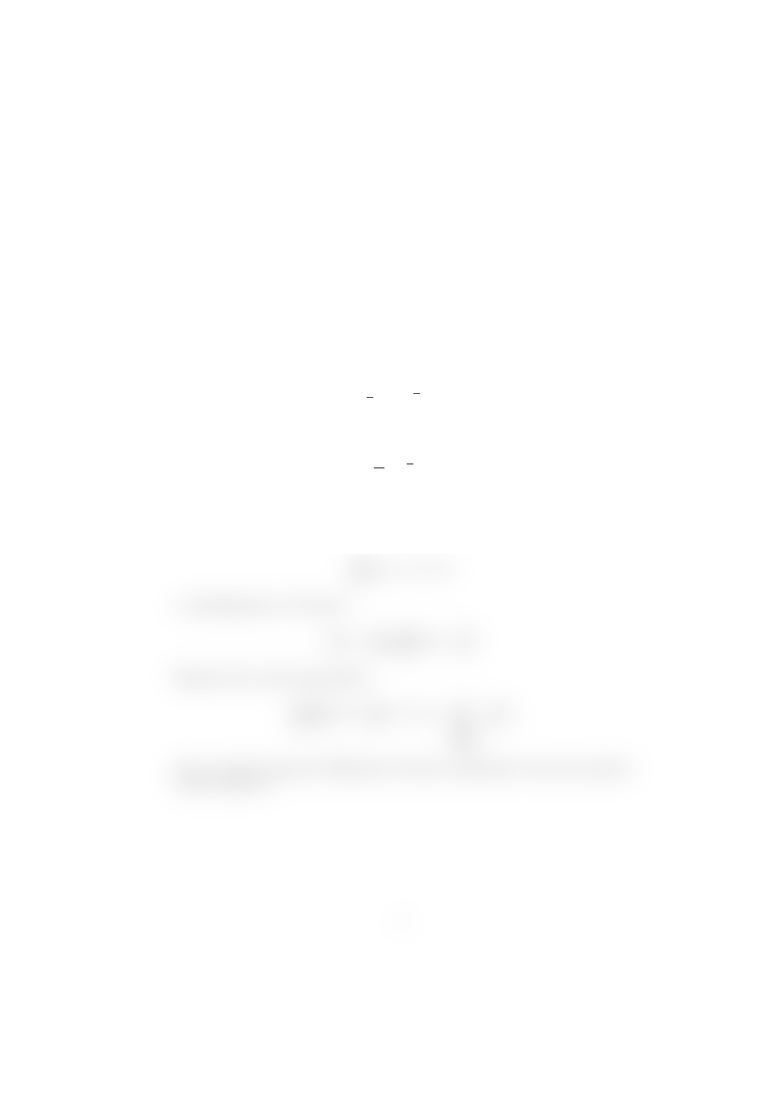Class Notes (1,100,000)
CA (630,000)
SFU (10,000)
MATH (300)
Lecture

# Integral formulas for area

Department
Mathematics
Course Code
MATH 322
Professor
John Michael Stockie

This preview shows half of the first page. to view the full 1 pages of the document.Integral formulas for area
November 29, 2011
During class, I gave the following formula for calculating the area Ainside
a closed, positively-oriented, contour C:
1
2
Im
I
C
z dz. (1)
The text gives the following formula in Exercise 7, page 163:
1
2i
I
C
z dz. (2)
This seems confusing. Which formula is correct?
Well, I derived Equation (1) in class, and so it must be correct. To see what’s
wrong with Equation (2), let’s split the integral into its real and imaginary parts
I
C
z dz =X+iY,
so that Equation (1) becomes
Area =
1
2
Im
I
C
z dz =
1
2
Y.
Equation (2) on the other hand is
1
2i
I
C
z dz =
1
2i
(X+iY ) =
1
2
Y
|{z}
Area
i
2
X,
So it is only the real part of Equation (2) that corresponds to the area enclosed
by the contour C.
1